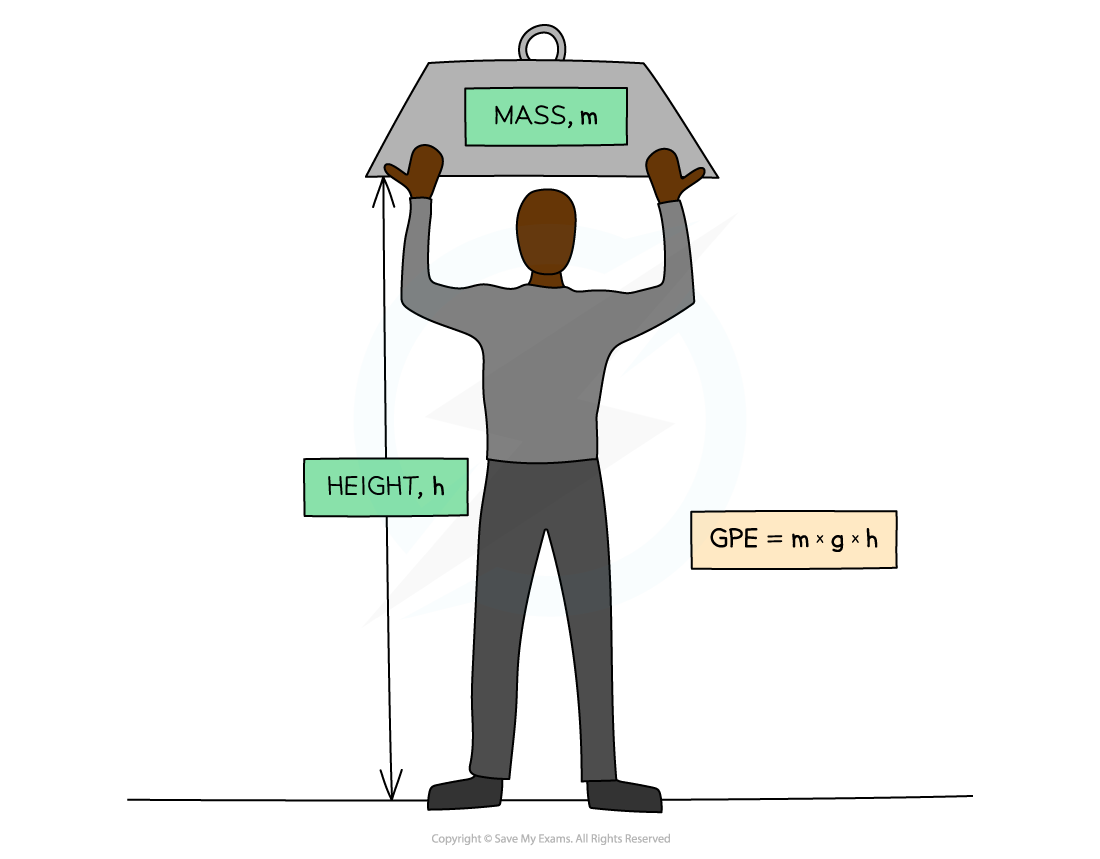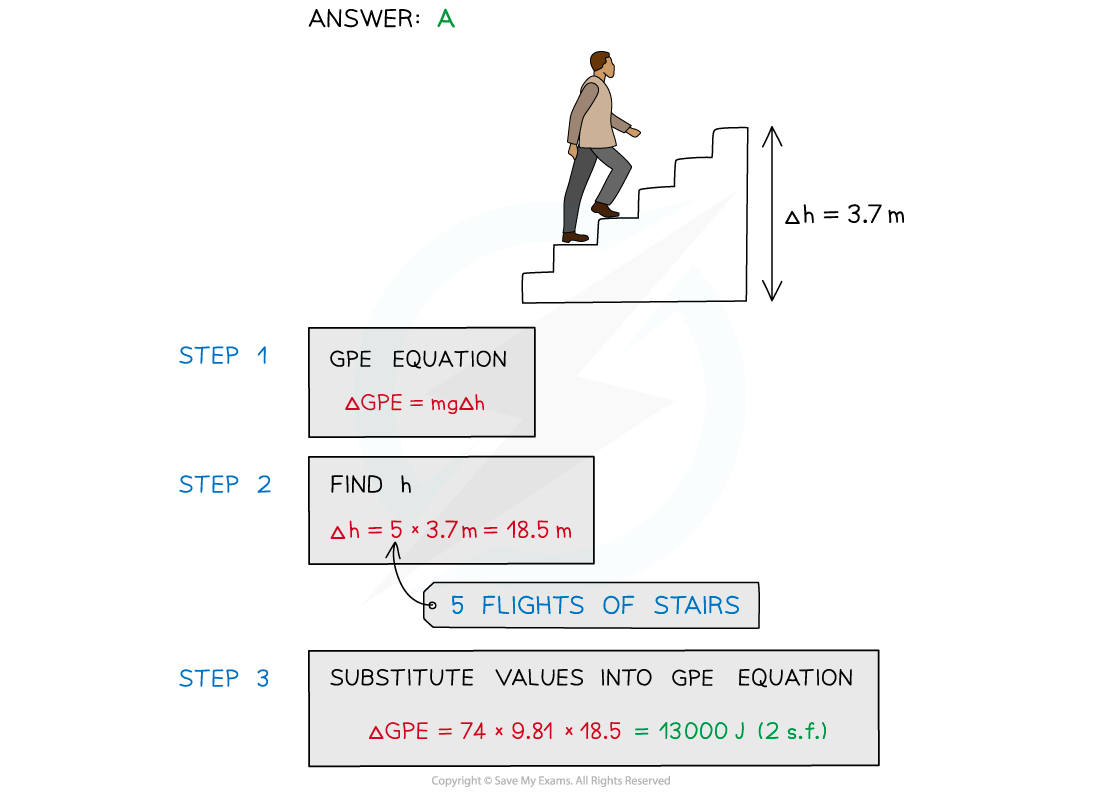# AQA A Level Physics复习笔记4.6.5 Kinetic & Gravitational Potential Energy

### Kinetic & Gravitational Potential Energy

#### Kinetic Energy

• Kinetic energy is the energy an object has due to its motion (or velocity)
• The faster an object is moving, the greater its kinetic energy

• When an object is falling, it is gaining kinetic energy since it is gaining speed
• This energy transferred from the gravitational potential energy it is losing

• An object will maintain this kinetic energy unless its speed changesKinetic energy (KE): The energy an object has when its moving

#### Worked Example

A body travelling with a speed of 12 m s-1 has kinetic energy 1650 J.If the speed of the body is increased to 45 m s-1, what is its new kinetic energy?#### Gravitational Potential Energy

• Gravitational potential energy (GPE) is energy stored in a mass due to its position in a gravitational field
• If a mass is lifted up, it will gain GPE (converted from other forms of energy)
• If a mass falls, it will lose GPE (and be converted to other forms of energy)

• The equation for gravitational potential energy for energy changes in a uniform gravitational field is:Gravitational potential energy (GPE): The energy an object has when lifted up

• The potential energy on the Earth’s surface at ground level is taken to be equal to 0
• This equation is only relevant for energy changes in a uniform gravitational field (such as near the Earth’s surface)

#### GPE v Height Graphs

• The two graphs below show how GPE changes with height for a ball being thrown up in the air and when falling downGraphs showing the linear relationship between GPE and height

• Since the graphs are straight lines, GPE and height are said to have a linear relationship
• These graphs would be identical for GPE against time instead of height

#### Worked Example

To get to his apartment a man has to climb five flights of stairs.

The height of each flight is 3.7 m and the man has a mass of 74 kg.

What is the approximate gain in the man's gravitational potential energy during the climb?

A.     13 000 J               B.     2700 J               C.     1500 J               D.     12 500 J#### Exam Tip

GPE:

• This equation only works for objects close to the Earth’s surface where we can consider the gravitational field to be uniform.
• At A level, you might have to consider examples where the gravitational field is not uniform, such as in space, where this equation for GPE will not be relevant.

KE:

• When using the kinetic energy equation, note that only the speed is squared, not the mass or the ½.
• If a question asks about the ‘loss of kinetic energy’, remember not to include a negative sign since energy is a scalar quantity.